Previous Page | Next Page

 Working with Matrices

## Subscripts

Subscripts are special postfix operators placed in square brackets
after a matrix operand. Subscript operations have the following general form:
where
operand
is usually a matrix name, but it can also be an expression or literal.
row
refers to an expression, either scalar or vector, for selecting one or more rows from the operand.
column
refers to an expression, either scalar or vector, for selecting one or more columns from the operand.

You can use subscripts to do any of the following:

• refer to a single element of a matrix
• refer to an entire row or column of a matrix
• refer to any submatrix contained within a matrix
• perform a reduction across rows or columns of a matrix
In expressions, subscripts have the same (high) precedence as the transpose postfix operator (). Note that when both row and column subscripts are used, they are separated by a comma. If a matrix has row or column labels associated with it from a MATTRIB or READ statement, then the corresponding row or column subscript can be a character matrix whose elements match the labels of the rows or columns to be selected.

### Selecting a Single Element

You can select a single element of a matrix in several ways. You can use two subscripts (row, column) to refer to its location, or you can use one subscript to look for the element down the rows. For instance, referring to the coffee example used earlier, find the element corresponding to the number of cups that Linda drank on Monday.

First, you can refer to the element by row and column location. In this case, you want the second row and first column. You can call this matrix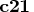. Here is the code:

```
>  print  coffee[rowname=names];

COFFEE
JENNY          4         2         2         3         2
LINDA          3         3         1         2         1
JIM            2         1         0         2         1
SAMUEL         5         4         4         3         4

>  c21=coffee[2,1];
>  print c21;

C21
3
```
You could also use row and column labels, which can be assigned with an MATTRIB statement as follows:
```
>  mattrib coffee rowname=names
>     colname={'MON' 'TUE' 'WED' 'THU' 'FRI'};

>  c21=coffee['LINDA','MON'];
>  print c21;

C21
3
```
You can also look for the element down the rows. In this case, you refer to this element as the sixth element of COFFEE in row-major order. Here is the code:
```
>  c6=coffee;
>  print c6;

C6
3
```

### Selecting a Row or Column

To refer to an entire row or column of a matrix, write the subscript with the row or column number, omitting the other subscript but not the comma. For example, to refer to the row of COFFEE that corresponds to Jim, you want the submatrix consisting of the third row and all columns. The following statements isolate and print this submatrix:
```
>  jim=coffee[3,];
>  print jim;

JIM
2         1         0         2         1
```
You could also use the row labels assigned previously with the MATTRIB statement as follows:
```
>  jim=coffee['JIM',];
>  print jim;

JIM
2         1         0         2         1
```
If you want the data for Friday, you know that the fifth column corresponds to Friday, so you want the submatrix consisting of the fifth column and all rows. The following statements isolate and print this submatrix:
```
>  friday=coffee[,5];
>  print friday;

FRIDAY
2
1
1
4
```
You could also use the previously assigned column labels as follows:
```
>  friday=coffee[,'FRI'];
>  print friday;

FRIDAY
2
1
1
4
```

### Submatrices

You refer to a submatrix by the specific rows and columns you want. Include within the brackets the rows you want, a comma, and the columns you want. For example, to create the submatrix of COFFEE consisting of the first and third rows and the second, third, and fifth columns, use the following statements:
```
>  submat1=coffee[{1 3},{2 3 5}];
>  print submat1;

SUBMAT1
2         2         2
1         0         1
```
The first vector, {1 3}, selects the rows, and the second vector, {2 3 5}, selects the columns. Alternately, you can create the vectors beforehand and supply their names as arguments by using the following statements:
```
>  rows={1 3};
>  cols={2 3 5};
>  submat1=coffee[rows,cols];
```
Similarly, you can use the previously assigned row and column labels as follows:
```
>  submat1=coffee[{'JENNY' 'JIM'},{'TUE' 'WED' 'FRI'}];
>  print submat1;

SUBMAT1
2         2         2
1         0         1

>  rows={'JENNY' 'JIM'};
>  cols={'TUE' 'WED' 'FRI'};
>  submat1=coffee[rows,cols];
```

You can use index vectors generated by the index creation operator (:) in subscripts to refer to successive rows or columns. For example, to select the first three rows and last three columns of COFFEE, use the following statements:

```
>  submat2=coffee[1:3,3:5];
>  print submat2;

SUBMAT2
2         3         2
1         2         1
0         2         1
```

Note that, in each example, the number in the first subscript defines the number of rows in the new matrix; the number in the second subscript defines the number of columns.

### Selecting Multiple Elements

All matrices in IML are stored in row-major order, so you can select multiple elements of a matrix by listing the position of the elements in the matrix. The elements in the first row have positions 1 through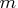, the elements in the second row have positions
through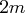, and the elements in the last row have positions
through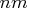.

For example, in the coffee example discussed previously, you might be interested in instances for which any person (on any day) drank more than two cups of coffee. The LOC function is useful for creating an index vector for a matrix that satisfies some condition. The following code uses the LOC function to find the desired instances:

```
>  h=loc( coffee > 2 );
>  print h;

H

1     4     6     7    16    17    18    19
```

The variable H contains indices of the COFFEE matrix. If you want to find the number of cups of coffee consumed on these occasions, you need to subscript the COFFEE matrix as follows:

```
>  cups = coffee[ h ];
>  print cups;

CUPS

4
3
3
3
5
4
4
3
4
```
IML always returns a column vector when a matrix is subscripted by a single array of positions. This might surprise you, but clearly the CUPS matrix cannot be the same shape as the COFFEE matrix since it contains a different number of elements. Therefore, the only reasonable alternatives are to return either a row vector or a column vector. Both would be valid choices, but IML returns a column vector.

Even if the original matrix is a row vector, the subscripted matrix will be a column vector, as the following example shows:

```
>  v = { -1 2 5 -2 7};  /* v is a row vector */
>  v2 = v[ {1 3 5} ];   /* v2 is a column vector */
>  print v2;

V2

-1
5
7
```

If you want to index into a row vector and want the resulting variable also to be a row vector, then use the following technique:

```
>  v = { -1 2 5 -2 7};  /* v is a row vector */
>  v2 = v[ ,{1 3 5} ];  /* Select columns. Note the comma. */
>  print v2;

V2

-1         5         7
```

### Subscripted Assignment

You can assign values into a matrix by using subscripts to refer to the element or submatrix. In this type of assignment, the subscripts appear on the left side of the equal sign. For example, to change the value in the first row, second column of COFFEE from 2 to 4, use subscripts to refer to the appropriate element in an assignment statement, as follows:
```
>  coffee[1,2]=4;
>  print coffee;

COFFEE
4         4         2         3         2
3         3         1         2         1
2         1         0         2         1
5         4         4         3         4
```
To change the values in the last column of COFFEE to zeros, use the following statements:

```
>  coffee[,5]={0,0,0,0};
>  print coffee;

COFFEE
4         4         2         3         0
3         3         1         2         0
2         1         0         2         0
5         4         4         3         0
```
As before, you could also use previously assigned row and column labels, as follows:
```
>  coffee[,'FRI']={0,0,0,0};
```
In the next example, you first locate the positions of negative elements of a matrix and then set these elements equal to 0. This can be useful in situations where negative elements might indicate errors or be impossible values. The LOC function is useful for creating an index vector for a matrix that satisfies some condition.

In the following statements, the LOC function is used to find the positions of the negative elements of the matrix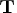and then to set these elements equal to 0 by using subscripted assignment:

```
>  t={ 3  2 -1,
>      6 -4  3,
>      2  2  2 };
>  print t;

T
3         2        -1
6        -4         3
2         2         2

>  i=loc(t<0);
>  print i;

I
3         5

>  t[i]=0;
>  print t;

T
3         2         0
6         0         3
2         2         2
```

Subscripts can also contain expressions with results that are either row or column vectors. These statements can also be written as follows:

```
>  t[loc(t<0)]=0;
```

If you use a noninteger value as a subscript, only the integer portion is used. Using a subscript value less than one or greater than the dimension of the matrix results in an error.Previous Page | Next Page | Top of Page